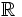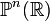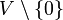# Real projective space

## Definition

### Short definition

Real projective space is defined as projective space over the field of real numbers$\R$, with its topological structure induced by the structure of the field of real numbers as a topological field.

### Finite-dimensional

Real projective space of dimension$n$, denoted$\R\mathbb{P}^n$ or$\mathbb{P}^n(\R)$, is defined as the quotient space under the group action$\R^{n+1} \setminus \{ 0 \}/\R^*$ where$\R^*$ acts by scalar multiplication. It is equipped with the quotient topology.

As a set, we can think of it as the set of lines through the origin in$\R^{n+1}$. Using an inner product on$\R^{n+1}$, it can also be identified with the set of hyperplanes of codimension 1 (i.e.,$n$-dimensional linear subspaces) in$\R^{n+1}$.

### Countable-dimensional

This space, called countable-dimensional real projective space and denoted$\R\mathbb{P}^\infty$, is defined as the quotient space of the nonzero elements of a countable-dimensional real vector space (with the standard topology) over$\R$ by the action of$\R^*$ by scalar multiplication.

### Others

We can also consider the real projective space corresponding to any topological real vector space, possibly infinite-dimensional, which is a real vector space equipped with a compatible topology. If the vector space is$V$, the projective space is defined as follows:we take$V \setminus \{ 0 \}$ with the subspace topology, and then put the quotient topology on its quotient under the action of$\R^*$.

## Particular cases$n$ Real projective space$\R\mathbb{P}^n$
0 one-point space
1 real projective line, which turns out to be homeomorphic to the circle
2 real projective plane
3 link: Fill this in later
countable ($\infty$) countable-dimensional real projective space

## Algebraic topology

### Homology

Further information: homology of real projective space

### Cohomology

Further information: cohomology of real projective space

### Homotopy

Further information: homotopy of real projective space